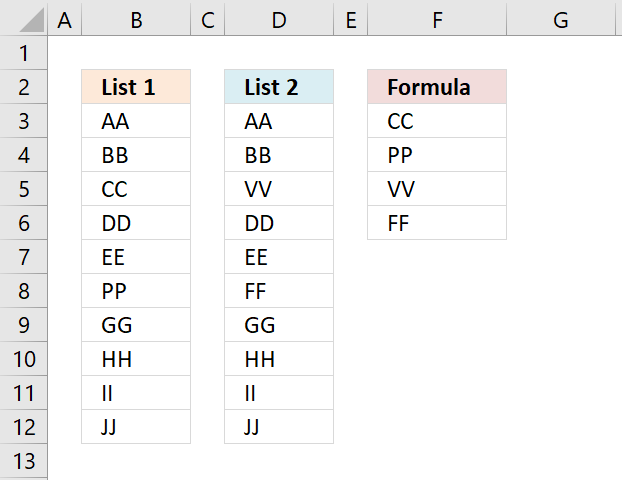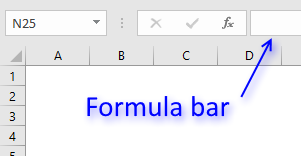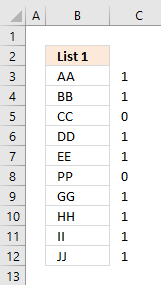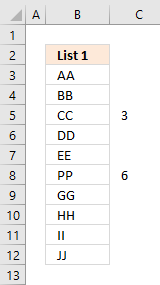Author: Oscar Cronquist Article last updated on February 03, 2019Question: How do i remove common values between two lists?

Answer: The solution in this article, removes common values and the original values, as seen on the picture above.

Array formula in cell F3:

=IFERROR(INDEX(\$B\$3:\$B\$12, SMALL(IF(COUNTIF(\$D\$3:\$D\$12,\$B\$3:\$B\$12)=0, MATCH(ROW(\$B\$3:\$B\$12),ROW(\$B\$3:\$B\$12)), ""), ROWS(\$A\$1:A1))), INDEX(\$D\$3:\$D\$12, SMALL(IF(COUNTIF(\$B\$3:\$B\$12,\$D\$3:\$D\$12)=0, MATCH(ROW(\$D\$3:\$D\$12),ROW(\$D\$3:\$D\$12)), ""), ROWS(\$A\$1:A1)-SUM((COUNTIF(\$D\$3:\$D\$12, \$B\$3:\$B\$12)=0)+0))))

To enter an array formula, type the formula in cell B3 then press and hold CTRL + SHIFT simultaneously, now press Enter once. Release all keys.The formula bar now shows the formula with a beginning and ending curly bracket telling you that you entered the formula successfully.

Don't enter the curly brackets yourself, they appear automatically.

### Explaining formula in cell F3

#### Step 1 - Count values

The COUNTIF function lets you count values based on a condition or criteria. COUNTIF(rangecriteria) The first argument is the cell range you want to evaluate.The criteria argument contains the values you want to look for.

COUNTIF(\$D\$3:\$D\$12,\$B\$3:\$B\$12)

becomes

COUNTIF({"AA"; "BB"; "VV"; "DD"; "EE"; "FF"; "GG"; "HH"; "II"; "JJ"},{"AA"; "BB"; "CC"; "DD"; "EE"; "PP"; "GG"; "HH"; "II"; "JJ"})

and returns the following array {1;1;0;1;1;0;1;1;1;1}.

The image to the right shows the array and the corresponding values, you can now easily see that CC and PP are not found in List 2.

#### Step 2 - Check if value is 0 (zero) and if so return the corresponding row number

If the array contains a zero the corresponding value is not found in the other list. The IF function lets you build a logical expression, if it evaluates to TRUE on thing happens and if FALSE another thing happens.IF(COUNTIF(\$D\$3:\$D\$12, \$B\$3:\$B\$12)=0, MATCH(ROW(\$B\$3:\$B\$12),ROW(\$B\$3:\$B\$12)), "")

becomes

IF({1;1;0;1;1;0;1;1;1;1}, {1;2;3;4;5;6;7;8;9;10}, "")

and returns the following array:

{"";"";3;"";"";6;"";"";"";""}

The image to the right shows the row numbers (relative to cell range \$B\$3:\$B\$12) in column C .

#### Step 3 - Extract the k-th smallest row number

The SMALL function returns the k-th smallest number from a cell range or an array. This allows us to extract a single value in a cell each.

SMALL(IF(COUNTIF(\$D\$3:\$D\$12, \$B\$3:\$B\$12)=0, MATCH(ROW(\$B\$3:\$B\$12),ROW(\$B\$3:\$B\$12)), ""), ROWS(\$A\$1:A1))

becomes

SMALL({"";"";3;"";"";6;"";"";"";""}, ROWS(\$A\$1:A1))

becomes

SMALL({"";"";3;"";"";6;"";"";"";""}, 1)

and returns 3.

#### Step 4 - Get the corresponding value

The INDEX function returns a value based on a row and column number, the column number is not necessary if the cell range only has one column.

INDEX(\$B\$3:\$B\$12, SMALL(IF(COUNTIF(\$D\$3:\$D\$12, \$B\$3:\$B\$12)=0, MATCH(ROW(\$B\$3:\$B\$12),ROW(\$B\$3:\$B\$12)), ""), ROWS(\$A\$1:A1)))

becomes

INDEX(\$B\$3:\$B\$12, 3)

and returns the value in cell B5 which is "CC" to cell F3.

#### Step 5 - Return values from List 2

When there are no more values to extract from List 1 the IFERROR function lets you continue with List 2.

The formula is repeated in the second argument except that other cell ranges are used. I am not going to explain these steps again, see above steps if you need to.

The fomula in cell F5 becomes

IFERROR(INDEX(\$B\$3:\$B\$12, SMALL(IF(COUNTIF(\$D\$3:\$D\$12, \$B\$3:\$B\$12)=0,ROW(\$B\$3:\$B\$12)-MIN(ROW(\$B\$3:\$B\$12))+1,""),ROW(B1))),INDEX(\$D\$3:\$D\$12, SMALL(IF(COUNTIF(\$B\$3:\$B\$12,\$D\$3:\$D\$12)=0, ROW(\$D\$3:\$D\$12)-MIN(ROW(\$D\$3:\$D\$12))+1, ""), ROW(B1)-SUM((COUNTIF(\$D\$3:\$D\$12,\$B\$3:\$B\$12)=0)+0))))

becomes

IFERROR(#NUM,INDEX(\$D\$3:\$D\$12, SMALL(IF(COUNTIF(\$B\$3:\$B\$12,\$D\$3:\$D\$12)=0, ROW(\$D\$3:\$D\$12)-MIN(ROW(\$D\$3:\$D\$12))+1, ""), ROW(B1)-SUM((COUNTIF(\$D\$3:\$D\$12,\$B\$3:\$B\$12)=0)+0))))

becomes

=IFERROR(#NUM,INDEX(\$D\$3:\$D\$12, 3))

and returns "VV" in cell F5.

### Download Excel *.xlsx file

remove-common-values-between-two-columns3.xlsx

To create a unique list from two columns or two cell ranges, check this article out: Create unique list from two columns